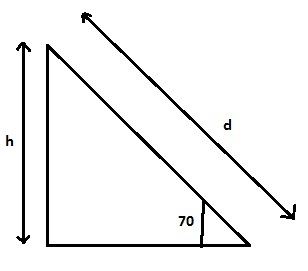# On a particular roller coaster, the first hill has a track length of 40 meters and makes an angle...

## Question:

On a particular roller coaster, the first hill has a track length of 40 meters and makes an angle of 70 degree with the ground. The cars (m = 500 kg) are pulled by a motor at a constant speed to the top of the hill.

(a) What is the change in the kinetic energy of the cars?

(b) What is the change in the potential energy of the cars?

(c) How much work is done by the motor?

## Principle of Energy Conservation

According to principle of energy conservation, energy can neither be created nor be destroyed but only can change its form. When an object doesn't change its speed then its kinetic energy remains constant. So any positive mechanical work on the object will change its potential energy if object is changing its height. The formula for potential and kinetic energy is as follows :

{eq}Potential \ energy = mgh \\ Kinetic \ energy = \frac{1}{2}mv^{2} {/eq}

Become a Study.com member to unlock this answer! Create your account• d = length of track = 40 m.
• h = vertical height of track = h.
• g = acceleration due to gravity = 9.8 {eq}m/s^{2} {/eq}.
• Speed of car is...Linear Momentum, Impulse & Energy Conservation

from

Chapter 49 / Lesson 1
5.1K

In this lesson, you'll learn about linear momentum, impulse, and energy conservation. These are all important components of collisions, which can be large, small, and everything in between!